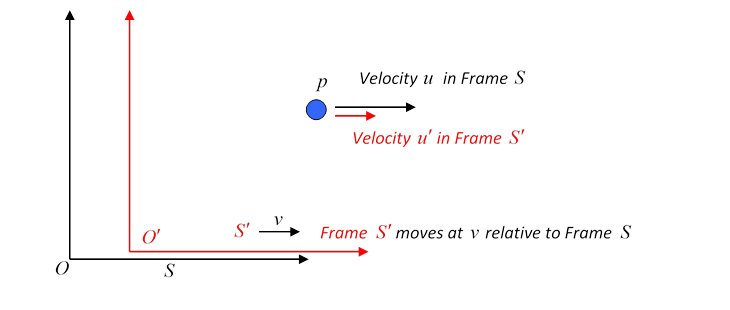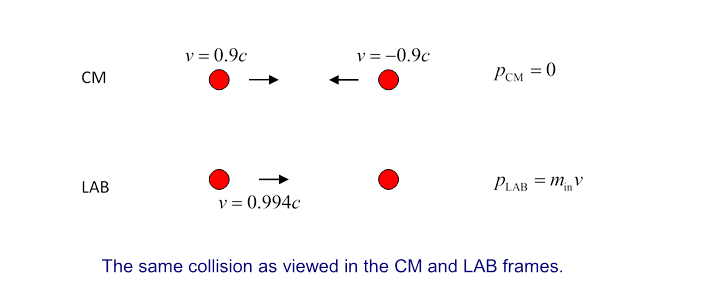# 63  Relativistic Dynamics

Michael Fowler

## Transforming Energy and Momentum to a New Frame

### An Invariant: the Rest Mass

We have shown (here) that

$\begin{array}{c}\stackrel{\to }{p}=m\stackrel{\to }{v}=\frac{{m}_{0}\stackrel{\to }{v}}{\sqrt{1-{v}^{2}/{c}^{2}}}\\ \\ E=m{c}^{2}=\sqrt{{m}_{0}^{2}{c}^{4}+{c}^{2}{\stackrel{\to }{p}}^{2}}.\end{array}$

Notice we can write this last equation in the form

${E}^{2}-{c}^{2}{\stackrel{\to }{p}}^{2}={m}_{0}^{2}{c}^{4}.$

That is to say, ${E}^{2}-{c}^{2}{\stackrel{\to }{p}}^{2}$ depends only on the rest mass of the particle and the speed of light.  It does not depend on the velocity of the particle, so it must be the same$—$for a particular particle$—$in all inertial frames.

This is reminiscent of the invariance of ${\stackrel{\to }{x}}^{2}-{c}^{2}{t}^{2},$ the interval between two events, under the Lorentz transformations.  One might guess from this that the laws governing the transformation from $E,p$ in one Lorentz frame to ${E}^{\prime },{p}^{\prime }$ in another are similar to those for  $t,x.$  We can actually derive the laws for $E,p$ to check this out.

As usual, we consider all velocities to be parallel to the $x$-axis.

We take the frame ${S}^{\prime }$ to be moving in the $x$ -direction at speed $v$ relative to $S.$Consider a particle of mass ${m}_{0}$ (rest mass) moving at ${u}^{\prime }$ in the ${x}^{\prime }$ direction in frame ${S}^{\prime },$ and hence at $u$ along $x$ in $S,$ where

$u=\frac{{u}^{\prime }+v}{1+v{u}^{\prime }/{c}^{2}}.$

The energy and momentum in ${S}^{\prime }$ are

${E}^{\prime }=\frac{{m}_{0}{c}^{2}}{\sqrt{1-{{u}^{\prime }}^{2}/{c}^{2}}},\text{ }\text{ }{p}^{\prime }=\frac{{m}_{0}{u}^{\prime }}{\sqrt{1-{{u}^{\prime }}^{2}/{c}^{2}}}$

and in $S:$

$E=\frac{{m}_{0}{c}^{2}}{\sqrt{1-{u}^{2}/{c}^{2}}},\text{ }\text{ }p=\frac{{m}_{0}u}{\sqrt{1-{u}^{2}/{c}^{2}}}.$

It is straightforward (and you should do it!) to show that

$E=\frac{{E}^{\prime }+v{p}^{\prime }}{\sqrt{1-{v}^{2}/{c}^{2}}}.$

Similarly, we can show that

$p=\frac{{p}^{\prime }+v{E}^{\prime }/{c}^{2}}{\sqrt{1-{v}^{2}/{c}^{2}}}.$

These are the Lorentz transformations for particle energy and momentum.

It follows that

${E}^{2}-{c}^{2}{p}^{2}={{E}^{\prime }}^{2}-{c}^{2}{{p}^{\prime }}^{2}={m}_{0}^{2}{c}^{4}.$

### Energy, Momentum and Four-Velocity

Reminder from the previous lecture: the four velocity on a world line is   ${U}^{\mu }=\frac{d{x}^{\mu }}{d\tau },$ in the nonrelativistic limit  $\left(c,{v}^{i}\right).$  The magnitude ${U}^{\mu }{U}_{\mu }=\frac{d{x}^{\mu }d{x}_{\mu }}{{\left(d\tau \right)}^{2}}=-{c}^{2}.$

In the rest frame, where the incremental movement along the world line $d{x}^{\mu }$ is purely in the time direction, and is just $cd\tau ,$ the four-velocity is $\left(c,0,0,0\right).$

In general, it's $\left(\gamma c,\gamma {v}^{1},\gamma {v}^{2},\gamma {v}^{3}\right).$  (Recall $dt=\gamma d\tau .$ )

Hence the energy (including rest energy) and momentum together can be written

$\left(E/c,\stackrel{\to }{p}\right)={m}_{0}{U}^{\mu },$

so $\left(E/c,\stackrel{\to }{p}\right)$ must transform just as $d{x}^{\mu }$ does.

### Photon Energies in Different Frames

For a zero rest mass particle, such as a photon, $E=cp,\text{ }{E}^{2}-{c}^{2}{p}^{2}=0$ in all frames.

Thus

$E=\frac{{E}^{\prime }+v{p}^{\prime }}{\sqrt{1-{v}^{2}/{c}^{2}}}=\frac{{E}^{\prime }+v{E}^{\prime }/c}{\sqrt{1-{v}^{2}/{c}^{2}}}={E}^{\prime }\sqrt{\frac{1+v/c}{1-v/c}}.$

Since $E=cp,\text{ }\text{ }{E}^{\prime }=c{p}^{\prime }$ we also have

$p={p}^{\prime }\sqrt{\frac{1+v/c}{1-v/c}}.$

Notice that the ratios of photon energies in the two frames coincides with the ratio of photon frequencies found in the Doppler shift$—$and this result is independent of quantum mechanics, we didn’t need to say photon, we could just have imagined a classical electromagnetic long wave packet, approximating a plane wave. And, thinking of a classical plane wave, as Jackson points out (page 529) the phase at some point must be the same as at the transformed point in a Lorentz transformation, since it’s just a number, counting from some origin. That is,

$\varphi =\omega t-\stackrel{\to }{k}\cdot \stackrel{\to }{x}={\omega }^{\prime }{t}^{\prime }-{\stackrel{\to }{k}}^{\prime }\cdot {\stackrel{\to }{x}}^{\prime }$

is invariant. This can only be true if $\left(\omega /c,\stackrel{\to }{k}\right)$ transforms in the same way as $\left({x}_{\alpha }\right)$, notice the down indices. Writing $\omega /c={k}_{0},$ this means (check the sign of $\beta$ from the low energy limit, or visualizing the wave)

$\begin{array}{l}{{k}^{\prime }}_{0}=\gamma \left({k}_{0}-\stackrel{\to }{\beta }\cdot \stackrel{\to }{k}\right)\\ {{k}^{\prime }}_{\parallel }=\gamma \left({k}_{\parallel }-\beta {k}_{0}\right)\\ {{k}^{\prime }}_{\perp }={k}_{\perp }.\end{array}$

This gives the familiar Doppler shift for light, ${\omega }^{\prime }=\gamma \omega \left(1-\beta \mathrm{cos}\theta \right),$ and in particular, in contrast to sound, there is even a Doppler shift (from $\gamma$ ) when $\theta =\pi /2.$

## Particle Creation: Energy into Mass

### Energy Necessary to Produce a Pion

The first "new" particle created in accelerators was the pion:

$p+p\to p+p+{\pi }^{0},$

and

$p+p\to p+n+{\pi }^{+}.$

The neutral pion mass is 135 MeV, the charged pions have mass 140 MeV, where we follow standard high energy practice in calling mc2 the “mass”.

An incoming proton with 135 MeV of kinetic energy will not be able to create a neutral pion (rest mass 135 MeV) in a collision with a stationary proton.  (Must also conserve momentum.)

Go to the center of mass frame, where initially two protons are moving towards each other with equal and opposite velocities, there being no total momentum.  Obviously, in this frame the least possible K.E. must be just enough to create the ${\pi }^{0}$ with all the final state particles $\left(p,p,{\pi }^{0}\right)$ at rest.

$E=\frac{2{m}_{p}{c}^{2}}{\sqrt{1-{v}^{2}/{c}^{2}}}=2{m}_{p}{c}^{2}+{m}_{\pi }{c}^{2}.$

we find the two incoming protons must both be traveling at 0.36c

In the lab frame we must add a velocity of 0.36c + 0.36c = 0.64c.  incoming proton has a relativistic mass of 1.3 times its rest mass, and thus a K.E. around 280 MeV.

To create a pion of rest energy 135 MeV, it is necessary to give the incoming proton at least 290 MeV of kinetic energy.  This is called the “threshold energy”.

### Antiproton Production

$p+p\to p+p+p+\overline{p}.$

$p+p\to p+p+{\pi }^{+}+\overline{p}$?

Doesn’t happen$—$so charge conservation isn’t the only constraint on which particles can be produced.  Experimentally, baryon number is also conserved.

### A Machine Built to Produce One Particle

One of the first modern accelerators, built at Berkeley in the fifties, was designed specifically to produce the antiproton.

We can use ${E}^{2}-{c}^{2}{\stackrel{\to }{p}}^{2}={{E}^{\prime }}^{2}-{c}^{2}{\stackrel{\to }{{p}^{\prime }}}^{2}$ to get lab frame information from the center of mass frame.

In the center of mass (CM) frame the momentum is zero, and in the lab frame the momentum is all in the incoming proton, so

${E}_{\text{cm}}^{2}={\left(\left({m}_{\text{in}}+{m}_{0}\right){c}^{2}\right)}^{2}-{c}^{2}{p}_{\text{in}}^{\text{2}}$

where here m0 is the proton rest mass, and

At the antiproton production threshold, Ecm = 4m0c2, so

$16{m}_{0}^{2}{c}^{4}={m}_{\text{in}}^{2}{c}^{4}+2{m}_{\text{in}}{c}^{2}{m}_{0}{c}^{2}+{m}_{0}^{2}{c}^{4}-{c}^{2}{p}_{\text{in}}^{2},$

and using

${m}_{\text{in}}^{\text{2}}{c}^{4}-{c}^{2}{p}_{\text{in}}^{\text{2}}={m}_{0}^{2}{c}^{4},$

we find

$2\left({m}_{\text{in}}{c}^{2}\right)\left({m}_{0}{c}^{2}\right)+2{\left({m}_{0}{c}^{2}\right)}^{2}=16{\left({m}_{0}{c}^{2}\right)}^{2}$,

so

${m}_{\text{in}}{c}^{2}=7{m}_{0}{c}^{2}$.

Therefore to create two extra particles, with total rest energy $2{m}_{0}{c}^{2},$ it is necessary for the incoming proton to have a kinetic energy of $6{m}_{0}{c}^{2}.$  The Berkeley Gevatron had design energy 6.2 GeV.

### Higher Energies

As we go to higher energies, this “inefficiency” gets worse$—$consider energies such that the kinetic energy >> rest energy, and assume the incoming particle and the target particle have the same rest mass, ${m}_{0},$ with the incoming particle having relativistic mass ${m}_{\text{in}}:$Comparing the center of mass energy with the lab energy at these high energies,

$\begin{array}{c}{E}_{\text{LAB}}=\left({m}_{\text{in}}+{m}_{0}\right){c}^{2},\\ {E}_{\text{CM}}^{\text{2}}={E}_{\text{LAB}}^{\text{2}}-{p}_{\text{LAB}}^{\text{2}}{c}^{2}\\ ={m}_{\text{in}}^{2}{c}^{4}+2{m}_{\text{in}}{c}^{2}{m}_{0}{c}^{2}+{m}_{0}^{2}{c}^{4}-{p}_{\text{LAB}}^{\text{2}}{c}^{2}\\ =2{m}_{0}{c}^{2}\left({m}_{\text{in}}{c}^{2}+{m}_{0}{c}^{2}\right).\end{array}$

For $m\gg {m}_{0},$

${E}_{CM}^{2}\approx 2{m}_{0}{c}^{2}m{c}^{2}\approx 2{m}_{0}{c}^{2}.{E}_{LAB}$

so

${E}_{CM}\approx \sqrt{2{m}_{0}{c}^{2}.{E}_{LAB}}$,

ultimately one must quadruple the lab energy to double the center of mass energy. So at higher energies, things get steadily worse$—$this is why colliders were built!